#2019 iT 邦幫忙鐵人賽DAY 2
2
AI & Data

## Day02 Geopandas初探

`Pandas`是資料分析熱門且好用的工具，相信熟悉資料分析的各路英雄好漢都不陌生

## Geopandas介紹

• shapely
• fiona
• descartes
• matplotlib
• pyproj
• numpy
..more

``````conda install -c conda-forge geopandas
``````

``````pip install git+git://github.com/geopandas/geopandas.git
``````

## 讀寫GIS資料

[Day 6] 常見的GIS資料格式 - iT 邦幫忙::一起幫忙解決難題，拯救 IT 人的一天

``````import geopandas as gpd
gdf_Rail=gpd.read_file('data/Rail/Rail.shp',encoding='utf-8')
gdf_Rail
``````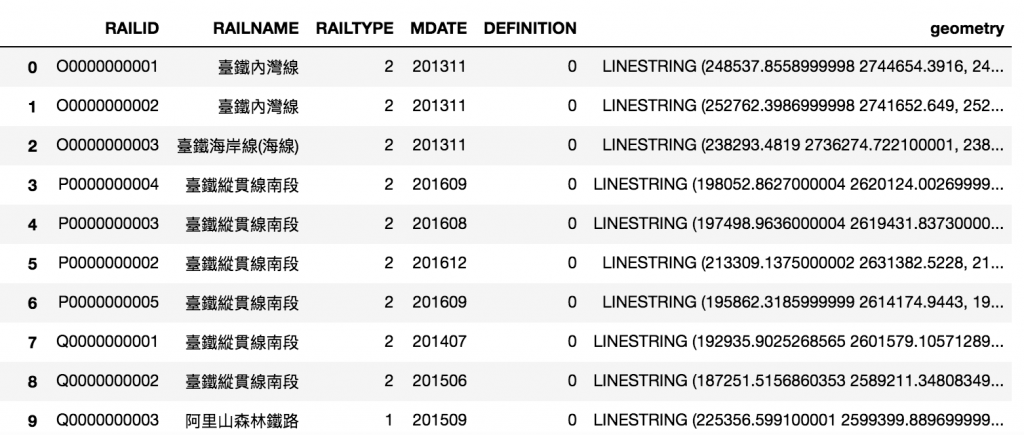## GeoDataFrame

GeoDataFrame與DataFrame類似，在[官方文件]中對於DataFrame的基本類型有一些說明。

## GeoDataFrame的操作

GeoDataFrame的許多基本操作與Pandas的DataFrame很

``````subset=gdf_Rail['RAILNAME']
``````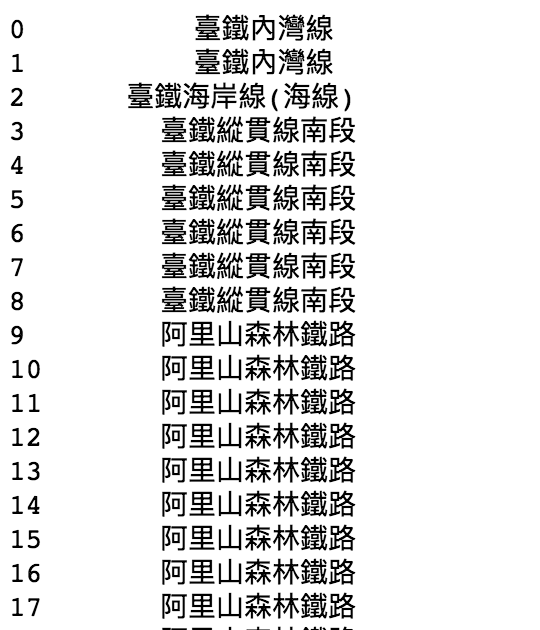subset again

``````subset=gdf_Rail.iloc[:3]
``````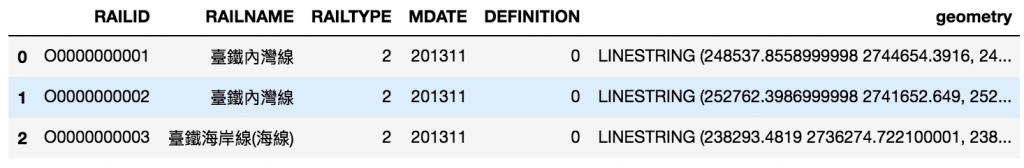``````sample=gdf_Rail.sort_values(by='MDATE')
``````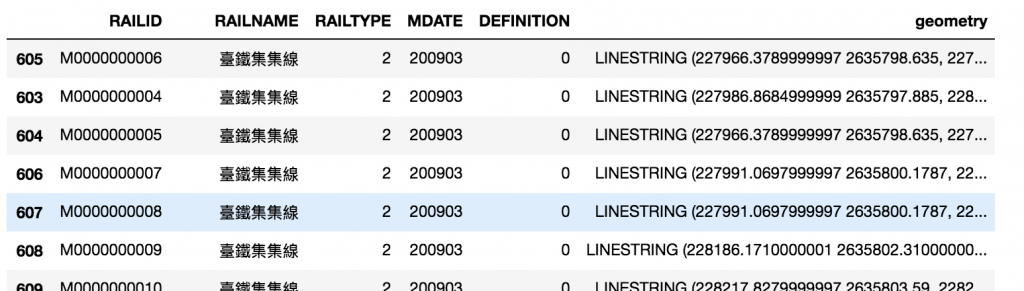``````sample.plot()
``````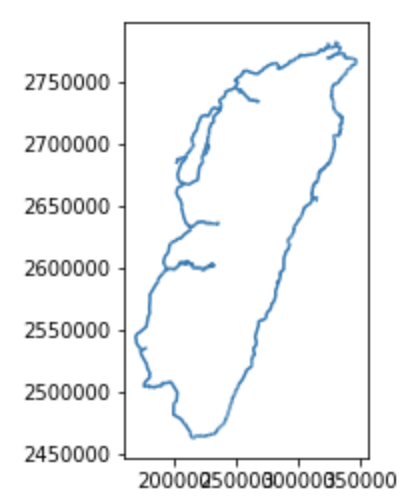``````sample.plot(column='RAILNAME')
``````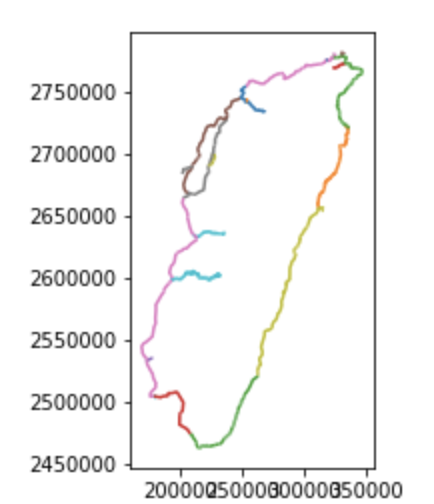GeoDataFrame讓GIS資料處理更方便，他與DataFrame操作體驗接近，今天也初探了一些簡單的功能

### 1 則留言

0

Chimin iT邦新手 5 級 ‧ 2020-04-30 10:33:23 檢舉

1.conda create --name myenv python=3.6
2.conda install -c conda-forge geopandas